## Content

### Functions and their inverses

We begin with a simple example.

#### Example

Let $$f(x) = 2x$$ and $$g(x) = \dfrac{x}{2}$$.

Apply the function $$g$$ to the number 3, and then apply $$f$$ to the result:

$g(3) = \dfrac{3}{2} \qquad\text{and}\qquad f\Bigl(\dfrac{3}{2}\Bigr) = 3.$

A similar thing happens if we first apply $$f$$ and then apply $$g$$:

$f(3) = 6 \qquad\text{and}\qquad g(6) = 3.$

It is clear that this will happen with any starting number. This is expressed as

\begin{align*} f(g(x)) &= x, \qquad\text{for all } x \\ g(f(x)) &= x, \qquad\text{for all } x. \end{align*}

The function $$f$$ reverses the effect of $$g$$, and the function $$g$$ reverses the effect of $$f$$. We say that $$f$$ and $$g$$ are inverses of each other.

As another example, we have

$(\sqrt{x})^3=x \qquad\text{and}\qquad \sqrt{x^3}=x,$

for all real $$x$$. So the functions $$f(x)=x^3$$ and $$g(x)=\sqrtx$$ are inverses of each other.

If $$x \geq 0$$, then $$(\sqrt{x})^2 = x$$ and $$\sqrt{x^2} = x$$. If $$x<0$$, then $$\sqrt{x}$$ is not defined. So the functions $$f(x)=x^2$$ and $$g(x)=\sqrt{x}$$ are inverses of each other, but we need to be careful about domains. We will look at this more carefully later in this section.

#### Basics

In an earlier section of this module, we defined the composite of two functions $$h$$ and $$g$$ by $$(g \circ h)(x)=g(h(x))$$.

###### Definitions
• The zero function $$\underline 0 \colon \mathbb{R} \to \mathbb{R}$$ is defined by $$\underline 0(x) = 0$$, for all $$x$$.
• The identity function $$\mathrm{id} \colon \mathbb{R} \to \mathbb{R}$$ is defined by $$\mathrm{id}(x) = x$$, for all $$x$$.

#### Example

Consider a function $$f \colon \mathbb{R} \to \mathbb{R}$$.

1. Prove that
1. $${\underline 0} \circ f = {\underline 0}$$
2. $$f \circ \mathrm{id} = f$$
3. $$\mathrm{id}\circ f = f$$.
2. Show that $$f\circ {\underline 0}$$ does not necessarily equal $$\underline 0$$.

#### Solution

1. We have $$({\underline 0}\circ f)(x) = {\underline 0}(f(x)) = 0$$, for all $$x$$, and so $${\underline 0}\circ f = {\underline 0}$$.
2. We have $$(f\circ \mathrm{id})(x) = f(\mathrm{id}(x)) = f(x)$$, for all $$x$$, and so $$f\circ \mathrm{id} = f$$.
3. We have $$(\mathrm{id}\circ f)(x) = \mathrm{id}(f(x)) = f(x)$$, for all $$x$$, and so $$\mathrm{id}\circ f = f$$.
1. Consider the function given by $$f(x)=2$$, for all $$x$$. Then $$f\circ {\underline 0}(x)=f({\underline 0}(x))=f(0)=2$$, and so $$f\circ {\underline 0}\neq {\underline 0}$$.
###### Definition

Let $$f$$ be a function with both domain and range all real numbers. Then the function $$g$$ is the inverse of $$f$$ if

\begin{align*} f(g(x)) &= x, \quad\text{for all } x, \quad\text{and} \\ g(f(x)) &= x, \quad\text{for all } x. \end{align*}

That is, $$f\circ g = \mathrm{id}$$ and $$g\circ f = \mathrm{id}$$.

###### Notes.
• Clearly, if $$g$$ is the inverse of $$f$$, then $$f$$ is the inverse of $$g$$.
• We denote the inverse of $$f$$ by $$f^{-1}$$. We read $$f^{-1}$$ as '$$f$$ inverse'. Note that $$f$$ inverse has nothing to do with the function $$\dfrac{1}{f}$$.

#### Example

Let $$f(x)=x+2$$ and let $$g(x)=x-2$$. Show that $$f$$ and $$g$$ are inverses of each other.

#### Solution

We have

$f(g(x)) = f(x-2)=x-2+2=x, \quad\text{for all } x\qquad (f\circ g = \mathrm{id})$

and

$g(f(x)) = g(x+2)=x+2-2=x, \quad\text{for all } x\qquad (g\circ f = \mathrm{id}).$

Hence, the functions $$f$$ and $$g$$ are inverses of each other.

##### Exercise 5

Find the inverse of

1. $$f(x) = x+7$$
2. $$f(x) = 4x+5$$.

#### Example

Let $$f(x)=ax+b$$ with $$a\neq 0$$. Find the inverse of $$f$$.

#### Solution

We have $$x = \dfrac{f(x)-b}{a}$$, for all $$x$$. So let $$g(x)=\dfrac{x-b}{a}$$. Then

\begin{align*} f(g(x)) &= f\Bigl(\dfrac{x-b}{a}\Bigr) = a\Bigl(\dfrac{x-b}{a}\Bigr)+b = x \\ g(f(x)) &= g(ax+b) = \dfrac{(ax+b)-b}{a} = x, \end{align*}

for all $$x$$. Hence, $$g$$ is the inverse of $$f$$.

##### Exercise 6
1. Show that $$f(x)=x^5$$ and $$g(x)=x^\frac{1}{5}$$ are inverses of each other.
2. Find the inverse of $$f(x)=x^3+2$$.

We do not yet have a general enough concept of inverses, since $$x^2$$ and $$\sqrt{x}$$ do not fit into this framework, nor do $$e^x$$ and $$\log_e x$$. We will give a definition that covers these functions later in this section.

#### The horizontal-line test

Consider the function $$f(x)=x^2$$, which has domain the reals and range $$A = \{\, x : x\geq 0 \,\}$$. Does $$f$$ have an inverse?

The following graph shows that it does not. We have $$f(-2) = f(2) = 4$$, and so $$f^{-1}(4)$$ would have to take two values, $$-2$$ and 2! Hence, $$f$$ does not have an inverse.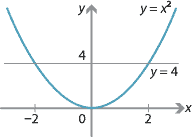This idea can be formulated as a test.

###### Horizontal-line test

Let $$f$$ be a function. If there is a horizontal line $$y = c$$ that meets the graph $$y = f(x)$$ at more than one point, then $$f$$ does not have an inverse.

Notes. Remember that the vertical-line test determines whether a relation is a function.

#### Example

Consider the function

$f(x) = x^3-x = (x+1)x(x-1).$

Its graph is shown in the following diagram.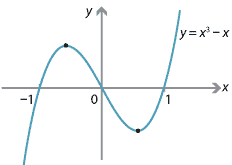Does $$f$$ have an inverse?

#### Solution

The line $$y=0$$ meets the graph at three points. By the horizontal-line test, the function $$f$$ does not have an inverse.

#### Finding inverses

Suppose that $$f(x)$$ and $$g(x)$$ are inverse functions. Then $$f(a)=b$$ if and only if $$g(b)=a$$. So $$(a,b)$$ is a point on the graph $$y=f(x)$$ if and only if $$(b,a)$$ is a point on the graph $$y=g(x)$$. The two points $$(a,b)$$ and $$(b,a)$$ are closely related, as the next theorem shows.

###### Theorem

The points $$P(a,b)$$ and $$Q(b,a)$$ are reflections of each other in the line $$y=x$$.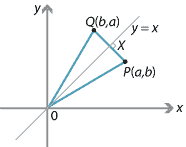###### Proof

Let $$X$$ be the point where $$PQ$$ meets the line $$y=x$$. Then

$\text{Gradient of } PQ = \dfrac{b-a}{a-b} = -1, \qquad \text{Gradient of } OX = 1,$

and so $$PQ$$ is perpendicular to $$OX$$. We also have $$OP^2 = a^2+b^2 = OQ^2$$, giving $$OP=OQ$$.

It now follows that $$\triangle OXP \equiv \triangle OXQ$$ (RHS), since $$OX$$ is a common side, $$OP = OQ$$ and $$OXP = 90^\circ = OXQ$$. Hence, $$XP = XQ$$.

We have shown that $$PQ\perp OX$$ and $$XP = XQ$$. So $$Q$$ is the reflection of $$P$$ in the line $$y = x$$, as required.

$$\Box$$

Notes.An alternative way to show that $$PX = QX$$ in this proof is by using the formula for the distance of a point from a line (see the module Coordinate geometry ). The formula gives $$PX = \dfrac{|b - a|}{\sqrt{2}} = QX$$.

###### Corollary

Assume that $$f$$ has an inverse. Then the graph of $$y=f^{-1}(x)$$ is the reflection of the graph of $$y=f(x)$$ in the line $$y=x$$.

We have seen that reflection in the line $$y=x$$ interchanges the points $$(a,b)$$ and $$(b,a)$$. So a function $$y=f(x)$$ is reflected to the relation $$x=f(y)$$. This is illustrated in the following diagram. A point $$(a,b)$$ on the graph $$y=f(x)$$ is mapped to the point $$(b,a)$$, which lies on $$x=f(y)$$.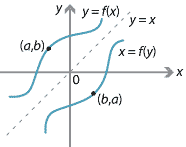Detailed description

This gives us a method for finding the inverse of $$y=f(x)$$ in some circumstances: Write down $$x=f(y)$$ and then, if possible, make $$y$$ the subject of this new equation.

For example, suppose $$y=4x+7$$. Swapping $$x$$ and $$y$$ yields

\begin{align*} x &= 4y+7 \\ 4y &= x-7 \\ y & =\dfrac{x-7}{4}. \end{align*}

Check that the functions $$f(x)=4x+7$$ and $$g(x)=\dfrac{x-7}{4}$$ are inverses of each other.

As a second example, suppose $$y=x^3+2$$. Swapping $$x$$ and $$y$$ yields

\begin{align*} x &= y^3+2 \\ y^3 &= x-2 \\ y &= \sqrt{x-2}. \end{align*}
##### Exercise 7

Consider the function $$f(x)=3x+2$$.

1. Find the inverse of $$f$$.
2. Show that the graph $$y=f(x)$$ meets the graph $$y=f^{-1}(x)$$ on the line $$y=x$$.
3. Draw a sketch showing $$y=f(x)$$ and $$y=f^{-1}(x)$$.

#### Increasing and decreasing functions

Consider two functions $$f_1$$ and $$f_2$$ with domain $$\mathbb{R}$$ and range $$\mathbb{R}$$. Assume that:

• $$f_1$$ is strictly increasing, that is, $$a < b \implies f_1(a) < f_1(b)$$
• $$f_2$$ is strictly decreasing, that is, $$a < b \implies f_2(a) > f_2(b)$$.

Then both $$f_1$$ and $$f_2$$ satisfy the horizontal-line test, and so they both have inverses. For example, the functions $$f_1$$ and $$f_2$$ could be those shown in the following diagram.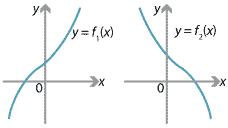###### Notes.
1. In the module Applications of differentiation , we see that:
• If $$f'(x)>0$$, for all $$x$$, then the function $$f$$ is strictly increasing.
• If $$f'(x)<0$$, for all $$x$$, then the function $$f$$ is strictly decreasing.

All such functions will satisfy the horizontal-line test.

1. It is easy to write down examples where it is not possible to give a formula for the inverse. For example, suppose $f(x) = x^5+3x^3+7x-8.$ Then $f'(x) = 5x^4+9x^2+7 \geq 7, \qquad\text{for all } x.$ Thus $$f$$ is a strictly increasing function, and hence $$f$$ has an inverse function. The graph of $$f$$ is $$y = x^5+3x^3+7x-8$$. If we want to find the inverse of $$f$$, we first interchange $$x$$ and $$y$$. This gives $x = y^5+3y^3+7y-8.$ From the look of this equation, it does not seem possible to make $$y$$ the subject, and indeed this the case. (There is a discussion of the solution of polynomial equations in the module Polynomials .) So we see that it is not always possible to explicitly write down a formula for the inverse, even when it exists.

#### Restricted domains and ranges

To deal with some of the simplest and most important examples, we have to generalise the idea of an inverse function by restricting the domain or the range or both.

For any set $$S$$, we shall use $$\mathrm{id}_S$$ to denote the identity function on $$S$$. That is, the function $$\mathrm{id}_S \colon S \to S$$ is given by $$\mathrm{id}_S(x) = x$$, for all $$x \in S$$.

#### Example

Define the set

$A = \{\, x\in\mathbb{R} : x\geq 0 \,\}.$

Then $$A$$ consists of all the positive reals together with 0. Now define the functions:

$f \colon A\to A,\quad f(x)=x^2 \qquad\text{and}\qquad g \colon A\to A,\quad g(x)=\sqrt{x}.$

Note that $$\mathrm{domain}(f) = \mathrm{range}(f) = A$$ and $$\mathrm{domain}(g) = \mathrm{range}(g) = A$$. We have

$(f\circ g)(x) = f(g(x)) = f(\sqrt{x}) = (\sqrt{x})^2 = x, \qquad\text{for all } x \in A,$

and so $$f\circ g = \mathrm{id}_A$$. Similarly, we can check that $$g\circ f = \mathrm{id}_A$$. It is natural to say

$g = f^{-1} \qquad\text{and}\qquad f = g^{-1}.$

Building on the previous example, we can also define functions $$h \colon A \to A$$ and $$j \colon A \to A$$ by $$h(x) = x^4$$ and $$j(x) = x^\dfrac{1}{4}$$, and we can show that $$h\circ j = j\circ h = \mathrm{id}_A$$. We say that $$j=h^{-1}$$ and $$h=j^{-1}$$.

There is no reason why this idea cannot be generalised to functions whose domain and range are not necessarily the same. We first give a definition which allows us to work in this situation.

###### Definition

Let $$f$$ be a function with domain $$X$$ and range $$Y$$. Then a function $$g$$ with domain $$Y$$ and range $$X$$ such that

$f\circ g = \mathrm{id}_Y \qquad\text{and}\qquad g\circ f = \mathrm{id}_X$

is the inverse of $$f$$. Clearly, if $$g$$ is the inverse of $$f$$, then $$f$$ is the inverse of $$g$$. We write $$g=f^{-1}$$ and $$f=g^{-1}$$.

#### Example

Let $$f \colon \mathbb{R} \to \mathbb{R}^+$$ be defined by $$f(x)=2^x$$, and let $$g \colon \mathbb{R}^+ \to \mathbb{R}$$ be defined by $$g(x)=\log_2 x$$.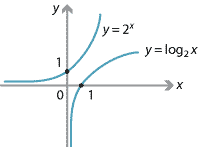Then

$(f\circ g)(x)=f(g(x))=f(\log_2 x)=2^{\log_2 x}=x, \qquad\text{for all } x>0.$

That is, $$f\circ g = i_{\mathbb{R}^+}$$. Also,

$(g\circ f)(x)=g(f(x))=g(2^x)=\log_2(2^x)=x, \qquad\text{for all } x\in \mathbb{R}.$

That is, $$g\circ f = i_{\mathbb{R}}$$. So $$f=g^{-1}$$ and $$g=f^{-1}$$.

#### Trigonometric functions

A scientific calculator reports that $$\sin^{-1}(0.5) = \dfrac{\pi}{6}$$. However, $$\sin(\dfrac{5\pi}{6}) = 0.5$$ as well. So why does $$\sin^{-1}(0.5)$$ not equal $$\dfrac{5\pi}{6}$$?

We start by considering the graph of $$y = \sin x$$.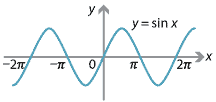This function spectacularly fails the horizontal-line test: whenever $$-1\leq c\leq 1$$, the line $$y=c$$ meets the graph $$y = \sin x$$ infinitely often!

To obtain an inverse function, we must restrict the domain of the function $$\sin x$$ so that, for each $$c$$ with $$-1\leq c\leq 1$$, there is exactly one value of $$x$$ with $$\sin x = c$$. Choice is involved, but clearly one would want to include the first quadrant $$[0,\dfrac{\pi}{2}]$$ in the domain, and to choose an interval if possible.

The natural choice is to define $$y = \sin x$$, for $$x \in [-\dfrac{\pi}{2}, \dfrac{\pi}{2}]$$. This function has an inverse, called $$\sin^{-1} x$$, with domain $$[-1,1]$$ and range $$[-\dfrac{\pi}{2}, \dfrac{\pi}{2}]$$. We have:

• $$\sin(\sin^{-1} x) = x$$, for all $$x$$ with $$-1\leq x\leq 1$$
• $$\sin^{-1}(\sin x) = x$$, for all $$x$$ with $$-\dfrac{\pi}{2} \leq x\leq \dfrac{\pi}{2}$$.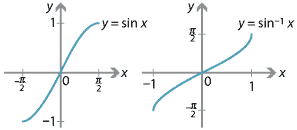Detailed description

In summary: $$\sin^{-1} a = b$$ if and only if $$\sin b = a$$, for $$a \in [-1,1]$$ and $$b \in [-\tfrac{\pi}{2}, \tfrac{\pi}{2}]$$.

##### Exercise 8
1. Work out how to restrict the domain of $$\cos x$$ and how to define $$\cos^{-1} x$$.
2. Repeat for $$\tan x$$.

Next page - Links forward - Differentiation